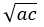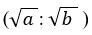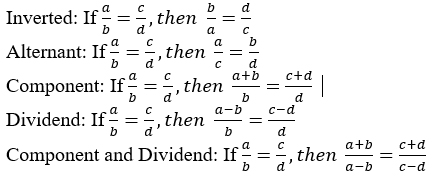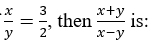# CBSE Worksheet for Chapter - 5 Ratio Proportion Class 8

## CBSE Worksheet For Class 8 Chapter - Ratio Proportion

1. The academic team of Physics Wallah prepared a worksheet for class 8 chapter ratio and proportion encourages the students to become familiar with many questions and think more about the topic. Questions are related to expressing ratios in a very simple way, simplifying ratios, comparing ratios, arranging ratios in ascending or descending order, and proportion and mean proportional between numbers. Do follow CBSE Worksheet for class 8 Mathematics. Check out main page of Physics Wallah.
2. Summary:
3. a, b, c, d are in proportion if a/d = b/c
4. Mean proportional:
5. If a: b:: b: c, then a/b = b/c
6. b2 = ac
b = √ac7. Duplicate Ratio: The duplicate ratio of (a : b) is (a2 : b2)
8. Sub-Duplicate Ratio: The sub-duplicate ratio of (a: b) is9. Triplicate Ratio: The Triplicate ratio of (a : b) is (a3 : b3)
10. Sub-Triplicate Ratio: The sub-triplicate ratio of (a: b) is (a1/3: b1/3)### Exercise:

1. If a: b = 5 : 9 and b : c = 4 : 7, find a : b : c.
2. Find the fourth proportional of 5, 8, 15.
3. If Rs. 782 be divided into three parts, proportional to 1/2:2/3:3/4 , then find the first part.
4. A bag contains 50 p, 25 p and 10 p coins in the ratio 5 : 9 : 4, amounting to Rs. 206. Find the number of coins of each type.
5. The third proportional of 0.36 and 0.48 is:
1. 0.64
2. 0.1728
3. 0.42
4. 0.94
6. If 2A = 3B and 4B = 5C, then A : C is:
1. 4 : 3
2. 8 : 15
3. 15 : 8
4. 3 : 4
7. If x : y = 5 : 2, then (8x + 9y) = (8x + 2y) is:
1. 22 : 29
2. 26 : 61
3. 29 : 22
4. 61 : 26
8. Two numbers are in the ratio 3 : 5. If 9 is subtracted from each, the new numbers are in the ratio 12 : 23. The smaller number is:
1. 27
2. 33
3. 49
4. 55
9. The ratio of three numbers is 3 : 4 : 7 and their product is 18144. The numbers are:
1. 9, 12, 21
2. 15, 20, 25
3. 18, 24, 42
4. none of these
10. What least common number must be subtracted from each of the numbers 14, 17, 34 and 42 so that the remainders may be proportional?
1. 0
2. 1
3. 2
4. 7
11. The speeds of three cars are in the ratio 5 : 4 : 6. The ratio between the time taken by them to travel the same distance is:
1. 5:4:6
2. 6:4:5
3. 10:12:15
4. 12:15:10
12. Monthly incomes of A and B are in the ratio of 4:3 and their savings are in the ratio of 3:2. If the expenditure of each will be Rs. 600, what will be the monthly income of B?
1. 2400
2. 1170
3. 1500
4. 1800
13. In a bag there are coins of 25p, 10p and 5p in the ratio of 1:2:3. If there is Rs.30 in all, how many 5p coins are there?
1. 50
2. 100
3. 150
4. 200
14. In a 60 liter mixture of milk and water, the ratio of milk to water is 2:1. How many liters of water is to be added to this, to make the ratio 1:2 in the new mixture?
1. 42
2. 56
3. 60
4. 70
15. x/y = 3/2 If , thenis:
1. 4/3
2. 1/2
3. 5/4
4. 5

### Solutions of Worksheet - 5 Chapter - Ratio Proportion

1. 20: 36: 63
2. 24
3. 204
4. 50p = 200 ; 25p = 360 ; 10p =160
5. A)
6. (C)
7. (C)
8. (B)
9. (C)
10. (C)
11. (D)
12. (D)
13. (C)
14. (C)
15. (D)